## Sample Test Problems

1. The relativistic correction to the Hydrogen Hamiltonian is. Assume that electrons have spin zero and that there is therefore no spin orbit correction. Calculate the energy shifts and draw an energy diagram for the n=3 states of Hydrogen. You may use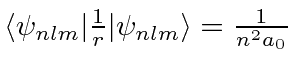and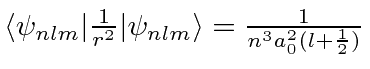.

2. Calculate the fine structure energy shifts (in eV!) for the,, and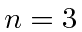states of Hydrogen. Include the effects of relativistic corrections, the spin-orbit interaction, and the so-called Darwin term (due to Dirac equation). Do not include hyperfine splitting or the effects of an external magnetic field. (Note: I am not asking you to derive the equations.) Clearly list the states in spectroscopic notation and make a diagram showing the allowed electric dipole decays of these states.

3. Calculate and show the splitting of thestates (as in the previous problem) in a weak magnetic field B. Draw a diagram showing the states before and after the field is applied

4. If the general form of the spin-orbit coupling for a particle of massand spin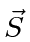moving in a potentialis, what is the effect of that coupling on the spectrum of an electron bound in a 3D harmonic oscillator? Give the energy shifts and and draw a diagram for theandstates.for the,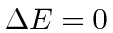,
for the,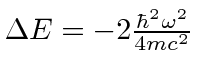,
for the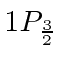,.
5. We computed that the energies after the fine structure corrections to the hydrogen spectrum are. Now a weak magnetic fieldis applied to hydrogen atoms in thestate. Calculate the energies of all thestates (ignoring hyperfine effects). Draw an energy level diagram, showing the quantum numbers of the states and the energy splittings.
6. In Hydrogen, thestate is split by fine structure corrections into states of definite,,, and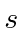. According to our calculations of the fine structure, the energy only depends on. We label these states in spectroscopic notation:. Draw an energy diagram for thestates, labeling each state in spectroscopic notation. Give the energy shift due to the fine structure corrections in units of.
7. The energies of photons emitted in the Hydrogen atom transition between the 3S and the 2P states are measured, first with no external field, then, with the atoms in a uniform magnetic field B. Explain in detail the spectrum of photons before and after the field is applied. Be sure to give an expression for any relevant energy differences.

Jim Branson 2013-04-22